# Divisibility Tests 2-12

## Divisibility Tests:

A number is divisible by 2 if its last digit is even (0,2,4,6 or 8)

A number is divisible by 3 if the sum of its digits is divisible by 3.

A number is divisible by 4 if the number's last two digits are divisible by 4.

A number is divisible by 5 if its last digit is a 0 or 5.

A number is divisible by 6 if it is divisible by 2 and 3 (see rules above).

There is no simple test for divisibility by seven, you need to do the division and check there is no remainder!

A number is divisible by 8 if the last three digits form a number that is divisible 8.

A number is divisible by 9 if the sum of the digits is divisible by 9.

A number is divisible by 10 if its last digit is 0.

A number is divisible by 11 if the alternating sum of its digits is divisible by 11. Alternating sum means a-b+c-d+... – m

A number is divisible by 12 if it is divisible by 3 and 4.

There is a printable worksheet to go with this activity.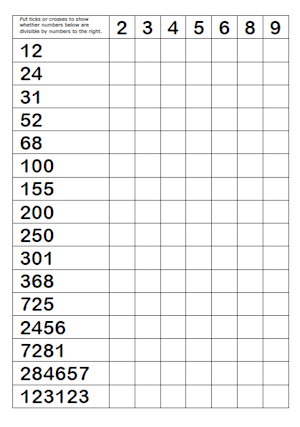#### Divisibility Test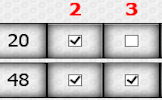Practise using the quick ways to spot whether a number is divisible by the digits two to nine.

The short web address is:

Transum.org/go/?to=divisibility

#### Divides Exactly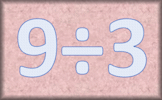Find which divisions result in whole number quotients. A mental arithmetic speed challenge.

The short web address is:

Transum.org/go/?to=dividesexactly

#### Divisibility Tests Worksheet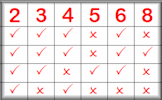This worksheet contains a list of the divisibility tests along with a fill-in-the-table exercise.

The short web address is:

Transum.org/go/?to=divtestprint

#### Delightfully Divisible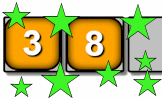Arrange the digits one to nine to make a number which is divisible in the way described.

The short web address is:

Transum.org/go/?to=delight

#### Bidmaze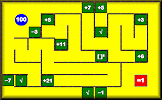Find your way through the maze encountering mathematical operations in the correct order to achieve the given total. This is an addictive challenge that begins easy but develops into quite a difficult puzzle.

This is a Shine+Write visual aid designed to be projected onto a whiteboard for whole class exposition.

You can find other popular visual aids on our Shine+Write page.

Find out about the latests developments on this website by signing up for a Transum Subscription. You will receive a regular newsletter and have access to the answers to most of the puzzles and activities on this site.

Transum,

Sunday, September 22, 2019

"The divisibility test for 7 is thanks to a 12-year old pupil, Chika Ofili, from Westminster School. You can read more about him here.

In a bored moment, Chika had turned his mind to finding a divisibility test for seven and this is what he came up with. He realised that if you take the last digit of any whole number, multiply it by 5 and then add this to the remaining part of the number, you will get a new number. And it turns out that if this new number is divisible by 7, then the original number is divisible by 7. What an easy test!

For example, take the number 532
53 + 2 x 5 = 63
63 is a multiple of 7, so 532 is divisible by 7

Well done Chika!"

#### Don't Shoot The Square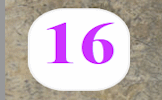You will need to be quick on the draw to shoot all of the numbers except the square numbers. So far this activity has been accessed 57806 times and 473 people have earned a Transum Trophy for completing it.For All:

©1997-2021 WWW.TRANSUM.ORG